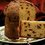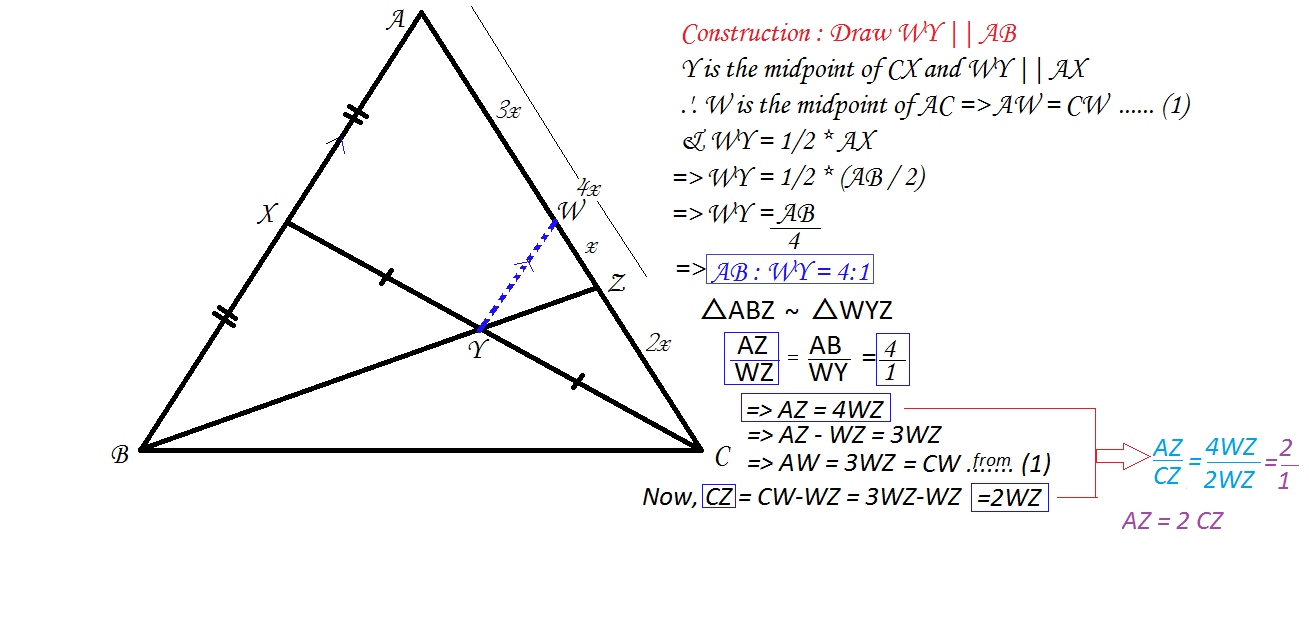# Geometry

Let $X$ be the midpoint of the side $AB$ of triangle $ABC$. Let $Y$ be the midpoint of $CX$. Let $BY$ cut $AC$ at $Z$. Prove that $AZ=2ZC$.Note by Subham Subian
4 years, 9 months ago

This discussion board is a place to discuss our Daily Challenges and the math and science related to those challenges. Explanations are more than just a solution — they should explain the steps and thinking strategies that you used to obtain the solution. Comments should further the discussion of math and science.

When posting on Brilliant:

• Use the emojis to react to an explanation, whether you're congratulating a job well done , or just really confused .
• Ask specific questions about the challenge or the steps in somebody's explanation. Well-posed questions can add a lot to the discussion, but posting "I don't understand!" doesn't help anyone.
• Try to contribute something new to the discussion, whether it is an extension, generalization or other idea related to the challenge.

MarkdownAppears as
*italics* or _italics_ italics
**bold** or __bold__ bold
- bulleted- list
• bulleted
• list
1. numbered2. list
1. numbered
2. list
Note: you must add a full line of space before and after lists for them to show up correctly
paragraph 1paragraph 2

paragraph 1

paragraph 2

[example link](https://brilliant.org)example link
> This is a quote
This is a quote
    # I indented these lines
# 4 spaces, and now they show
# up as a code block.

print "hello world"
# I indented these lines
# 4 spaces, and now they show
# up as a code block.

print "hello world"
MathAppears as
Remember to wrap math in $$ ... $$ or $ ... $ to ensure proper formatting.
2 \times 3 $2 \times 3$
2^{34} $2^{34}$
a_{i-1} $a_{i-1}$
\frac{2}{3} $\frac{2}{3}$
\sqrt{2} $\sqrt{2}$
\sum_{i=1}^3 $\sum_{i=1}^3$
\sin \theta $\sin \theta$
\boxed{123} $\boxed{123}$

Sort by:

We don't have to use cyclic properties.We extend the line $AY$ to meet $BC$ at $K.$We apply Menelaus theorem for $\triangle BCX$ where $AK$ is the transverse.So we get $\dfrac{BA}{AX}\times\dfrac{XY}{YC}\times\dfrac{KC}{KB}=-1.$Since $BA$ is twice of $AX$ and $XY=YC.$We get $\dfrac{KC}{KB}=\dfrac{1}{2}.$We don't have care about the minus sign as it only to show $A$ does not lie between $BX.$Next we apply Ceva's theorem for $\triangle ABC.$We get $\dfrac{AX}{BX}\times\dfrac{BK}{KC}\times\dfrac{ZC}{AZ}=1.$We know that $AX=BX$ and $BK=2KC.$So we get $\dfrac{ZC}{AZ}=\dfrac{1}{2}.$Therefore,$\boxed{AZ=2ZC}.$Hence proved.

- 4 years, 9 months ago

Why go for Menelaus when Thales' can do ?

- 4 years, 9 months ago

Proof:
Note that $ar(AXY)=ar (BXY)=ar(BYC)=ar(AYC)=\frac{ar(ABC)}{4}$
Since, median divides a triangle into 2 parts of equal areas.

Next, put AZ=$x$ and ZC=$y$
Now,
\frac {x}{x+y} * ar (ABC) = ar(ABZ) \(\implies \frac {x}{x+y}* ar (ABC) = \frac{ar (ABC)}{2} + ar (CYA)
$\implies \frac {x}{x+y} * ar (ABC) = \frac {ar (ABC}{2} + \frac{x}{x+y} * \frac {ar (ABC)}{4}$
$\implies \frac {x}{x+y}[ ar(ABC) - ar (CYA)] = ar(ABC)* \frac {1}{2}$
$\implies \frac {x}{x+y}[\frac {3}{4} * ar (CYAB) = \frac{ar (ABC)}{2}$
$\implies \frac {x}{x+y} * [\frac {3}{4}* ar (ABC)$
$\implies \frac {x}{x+y} = \frac {2}{3}$
$\implies x= 2y$
Hence, proved.

- 4 years, 9 months ago

Its not z, its x, unable to edit.
And forgive me for the brackets not being closed and other mistakes that I am unable to edit.

- 4 years, 9 months ago

I was unable to solve it using my limited knowledge of cyclic quads.

- 4 years, 9 months ago

There is no 3/4 in 4th last line.

- 4 years, 9 months ago- 4 years, 9 months ago

This question can easily be solved using midpoint theorem and similarity rules as above.

- 4 years, 9 months ago

Seriously, BC cut AC at Z; wont that make Z coincide with C and consequently CZ will be 0.

- 4 years, 9 months ago

sorry my bad .i corrected it plss try to solve it and give mme the solution i will be gratefull

- 4 years, 9 months ago

you can use the cevas theorem and the menuluas theorem to prove this

- 4 years, 9 months ago

and can use properties of cyclic quadrilaterals beacause my teacher said that it can be solve by using the properties of cyclic quadrilaterals

- 4 years, 9 months ago